# Mass - 4th grade - math problems

#### Number of problems found: 10

• Mother 8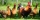Mother bought 2 kg of beef at P240 per kilo and 2 kg of chicken legs at P140 per kilo. She gave the meat vendor a P1000 bill. How much change did she get?
• Fruit importsCarriers import 18,324 kg of oranges from Spain. It was 16,857 kg less than mandarins and 2,645 kg more than bananas. a) how many kg did they bring mandarins? b) how many kg did bananas bring? c) how much total fruit?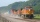The whole loaded train weighs 360 tons. It has twenty wagons, each carrying 12 tons of grain. What is the weight of the locomotive?
• Buns and toastsTwo buns weigh 10 grams more than two toasts. One Bun and two Toast weigh a total of 110 grams. How many grams weigh three toasts? How many grams does a Bun weigh?
• Flour2 kg of flour costs 100 CZK. How much does it cost half a kilogram?
• Farmers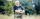Farmers loaded into a truck of fruit and vegetables intended for the store. 10 boxes of 5 kg pears, 8 boxes of 6 kg plums, 7 boxes 9 kg of carrots and 10 bags of 50 kg of potatoes. How many kilograms of fruit and vegetables loaded in total? How many kg co
• Locomotive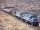The whole loaded train weighs 760 tons. It has twenty wagons, each carrying 20 tons of grain and empty weighs 10 tons. How much weight a locomotive?
• Grower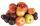Grower harvested 190 kg of apples. Pears harvested 10 times less. a) How many kg pears harvested? b) How many apples and pears harvested? c) How many kg harvested pears less than apples?
• Children in the classroom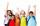How many children are in the classroom, where Ladislav is 10th lightest and 16th heaviest in class?
• Brick weightThe brick weighs 3 kg and a half-brick. How much does one brick weigh?

We apologize, but in this category are not a lot of examples.
Do you have an exciting math question or word problem that you can't solve? Ask a question or post a math problem, and we can try to solve it.

We will send a solution to your e-mail address. Solved examples are also published here. Please enter the e-mail correctly and check whether you don't have a full mailbox.

Mass - math problems. Examples for 4th grade.